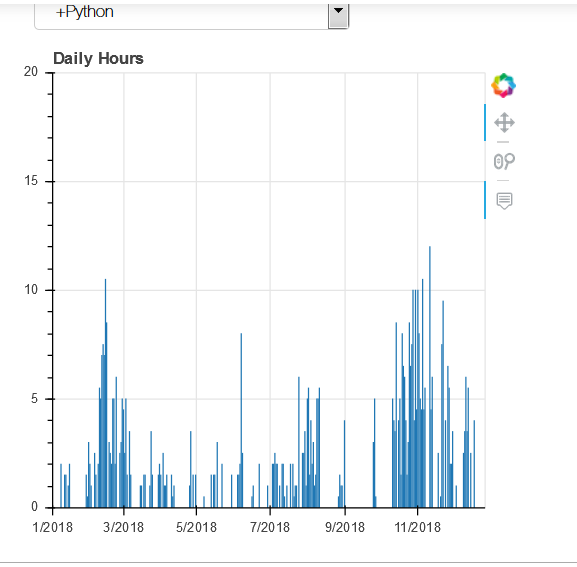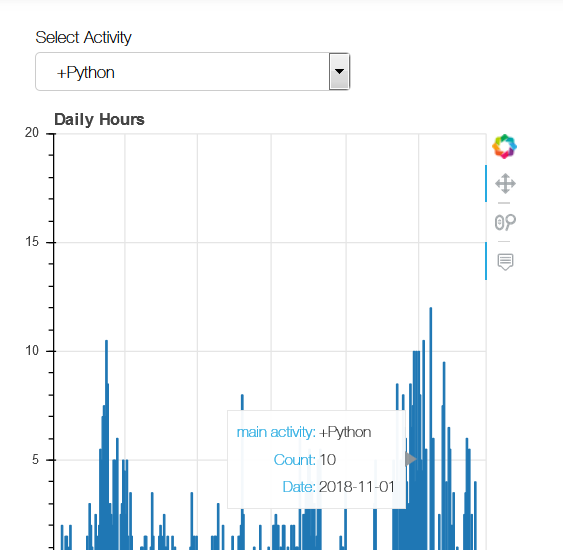# Minimum Vbar Width for hovering over it

I am creating a Vertical Bar based on my time records during the 2018.

I was creating a Vertical Bar

hover_2=HoverTool(tooltips=[(‘main activity’,’@main_activity’),(‘Count’,’@Count’),(‘Date’,’@Date{%F}’)], formatters={

‘Date’ : ‘datetime’})

plot=figure(title=‘Daily Hours’,tools=[hover_2,‘pan’,‘wheel_zoom’],plot_width=400, plot_height=400,x_range=(xrange), y_range=(yrange))

plot.vbar(x=“Date”, top=“Count”,source=source, width=1000000)

``

What I have discovered that in a time span of 365 days (1Y)

I can hover and get the required information only if the width is at least “1000000”

With Smaller Values I am not able to hover over the graph.

The first example is with a width value of 1.5.The second example is with a width value of 10000001. Why this kind of behavior?
2. How to know the minimal width?

Hi,

Thanks,

Bryan

···

On Feb 20, 2019, at 07:42, Andrea Ciufo <[email protected]> wrote:

I am creating a Vertical Bar based on my time records during the 2018.

I was creating a Vertical Bar
hover_2=HoverTool(tooltips=[('main activity','@main_activity'),('Count','@Count'),('Date','@Date{%F}')], formatters={
'Date' : 'datetime'})
plot=figure(title='Daily Hours',tools=[hover_2,'pan','wheel_zoom'],plot_width=400, plot_height=400,x_range=(xrange), y_range=(yrange))
plot.vbar(x="Date", top="Count",source=source, width=1000000)

What I have discovered that in a time span of 365 days (1Y)
I can hover and get the required information only if the width is at least "1000000"

With Smaller Values I am not able to hover over the graph.
The first example is with a width value of 1.5.
<1.5.PNG>

The second example is with a width value of 1000000

<10000Python.PNG>

• Why this kind of behavior?
• How to know the minimal width?

--
You received this message because you are subscribed to the Google Groups "Bokeh Discussion - Public" group.
To unsubscribe from this group and stop receiving emails from it, send an email to [email protected].
To post to this group, send email to [email protected].
To view this discussion on the web visit https://groups.google.com/a/continuum.io/d/msgid/bokeh/e9590e50-daf8-4a49-82c9-945092f1a340%40continuum.io.